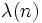# Universal exponent

This article defines an arithmetic function or number-theoretic function: a function from the natural numbers to a ring (usually, the ring of integers, rational numbers, real numbers, or complex numbers).
View a complete list of arithmetic functions

## Definition

Letbe a natural number. The universal exponent or Carmichael function of, denotedis defined in the following equivalent ways:

• It is the exponent of the multiplicative group modulo.
• It is the least common multiple of the orders, modulo, of all integers relatively prime to.
• It is the largest possible order, modulo, of an integer relatively prime to.

The symbolis also used for the Liouville lambda-function, which is totally different, while the capital letteris used for the von Mangoldt function, which is totally different too.